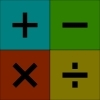#### You may also like### Worms

Place this "worm" on the 100 square and find the total of the four squares it covers. Keeping its head in the same place, what other totals can you make?### Which Scripts?

There are six numbers written in five different scripts. Can you sort out which is which?### Highest and Lowest

Put operations signs between the numbers 3 4 5 6 to make the highest possible number and lowest possible number.

##### Age 7 to 11Challenge Level
Congratulations to Cong, Katie and Charlie and also Josie, Dominic and George from St Nicolas CE Junior School, who all sent in correct solutions to this problem.

George has this to say on finding the larger of two fractions: What you have to do is look at the two fractions and look which is nearest to the right hand side of the wall. Then to find out by how much, you just look at the fraction wall and see which fraction will go in between the two fractions.

Katie's solution can be viewed by clicking here .

Charlie summarises the solution as follows:

$\frac{1}{3}$ is $\frac{2}{24}$ or $\frac{1}{12}$ bigger than $\frac{2}{8}$
$\frac{3}{4}$ is $\frac{1}{12}$ smaller than $\frac{5}{6}$
$\frac{1}{3}$ is $\frac{3}{6}$ or $\frac{1}{2}$ smaller than $\frac{5}{6}$
$\frac{3}{4}$ of $\frac{2}{3} = \frac{3}{4}$ of $\frac{8}{12 }= \frac{6}{12} = \frac{1}{2}$